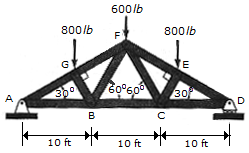### Create an Account

Home / Questions / What is type of this truss Determine the support reactions in A and B Determine the memb...

# What is type of this truss Determine the support reactions in A and B Determine the member forces in AB Determine the member forces in AG Determine the member forces in GF Determine theWhat is type of this truss?

Determine the support reactions in A and B.

Determine the member forces in AB.

Determine the member forces in AG.

Determine the member forces in GF.

Determine the member forces in EF.

Determine the member forces in DE.

Determine the member forces in FB.

Determine the member forces in BC.

Also determine the nature of the forces.compression or tension.

May 19 2020 View more View LessSubscribe To Get Solution